C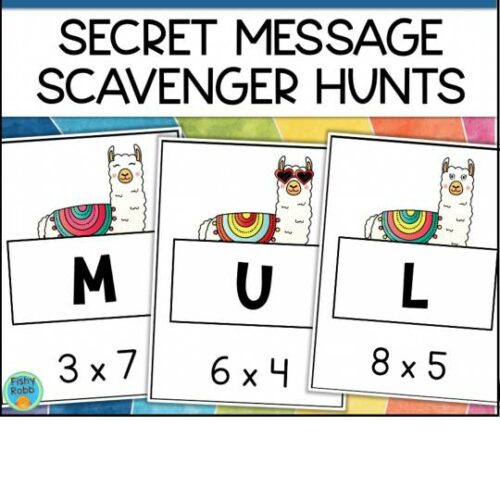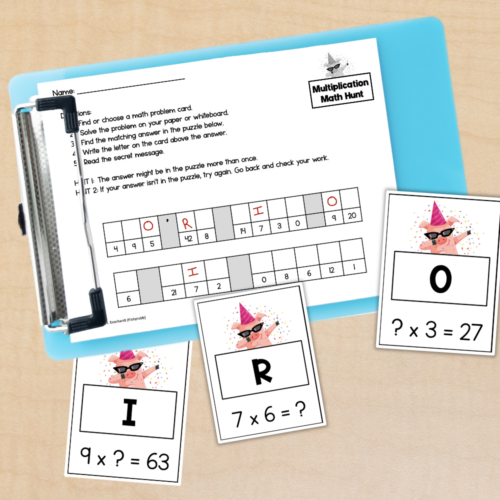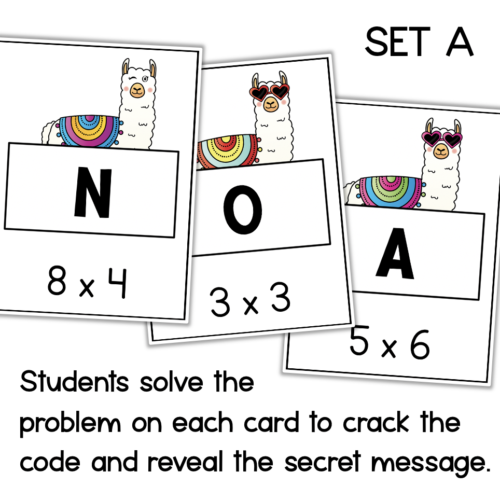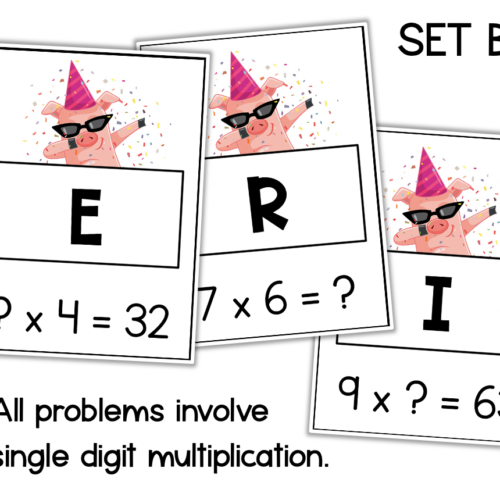### Details

Audience
Homeschool, Student, Teacher
3rd
Resource Type
Pages
14 Pages
Subject
Basic Math, Multiplication

### Description

This is a set of multiplication scavenger hunt activities that give student a fun way to practice multiplication facts and build math fluency.

To play, scatter the cards around the room. When students find a card, they solve the multiplication problem and fill in the letter on their code sheet. If all problems are solved correctly, a secret message will be revealed!

There are 2 separate scavenger hunts in this resource:
• Set A: Llama theme - Students solve to find the product.
• Set B: Pig theme - Students complete the multiplication equation by filling in the unknown number (missing factor or product).

Each set includes:
• 15-17 multiplication problem cards
• Secret code recording sheet

These activities can also be used in your math centers instead of as a scavenger hunt. Instructions included in the file.

This resource is aligned to the following math standards:

3.OA.A.4
Determine the unknown whole number in a multiplication or division equation relating three whole numbers. For example, determine the unknown number that makes the equation true in each of the equations 8 × ? = 48, 5 = __ ÷ 3, 6 × 6 = ?.

3.OA.C.7
Fluently multiply and divide within 100, using strategies such as the relationship between multiplication and division (e.g., knowing that 8 × 5 = 40, one knows 40 ÷ 5 = 8) or properties of operations. By the end of Grade 3, know from memory all products of two one-digit numbers.
This is a set of multiplication scavenger hunt activities that give student a fun way to practice multiplication facts and build math fluency. To play, scatter the cards around the room. When students find a card, they solve the multiplication... more

### Reviews

0
Overall review score

Displaying All Reviews | 0 Reviews

0
0%
0
0%
0
0%
0
0%
0
0%

### More from this shop5
(1)
\$4.00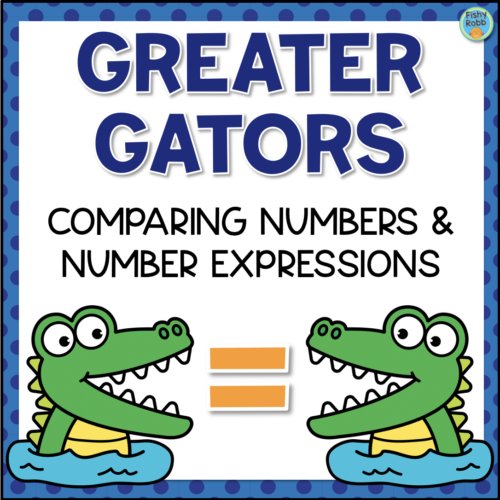5
(1)
\$3.95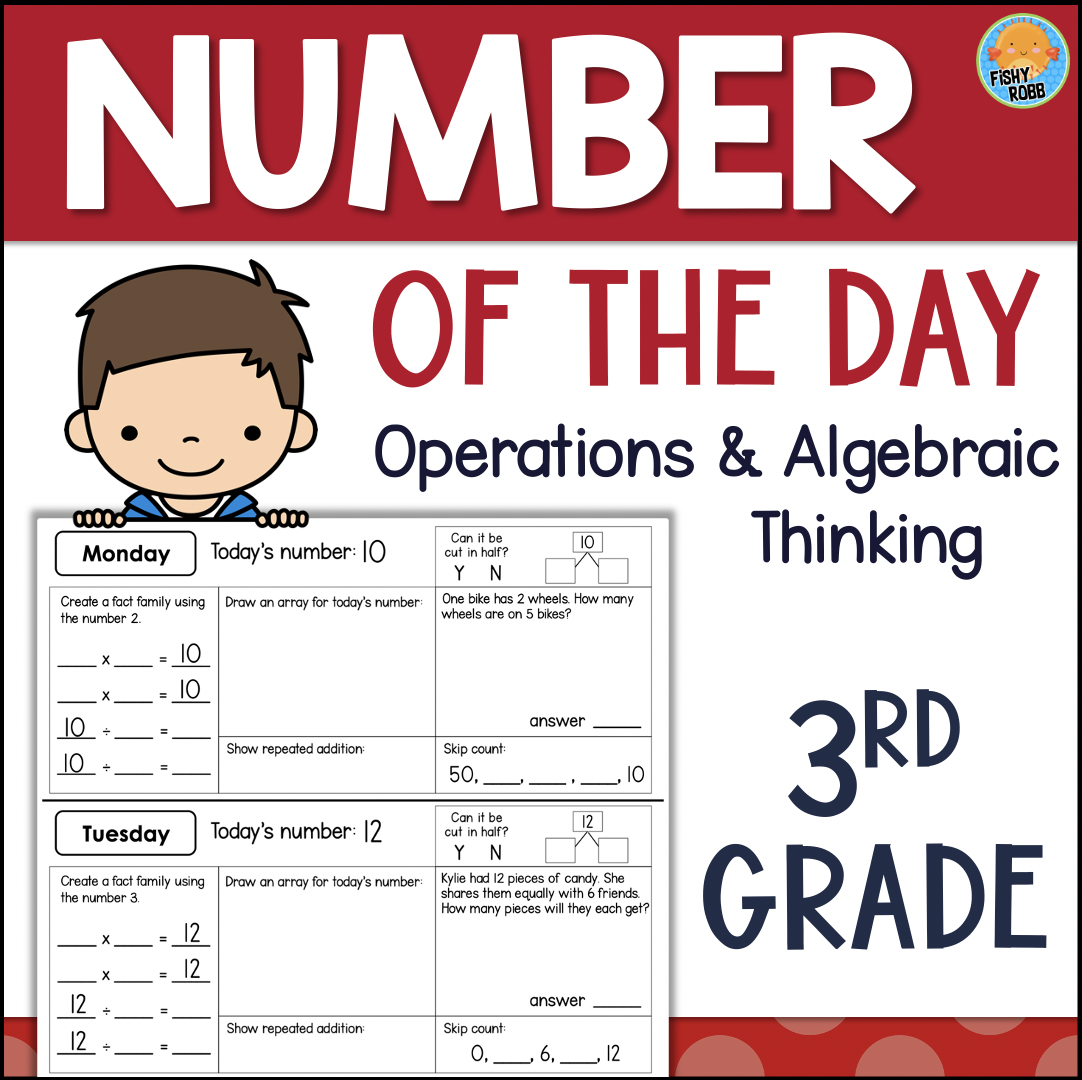\$3.75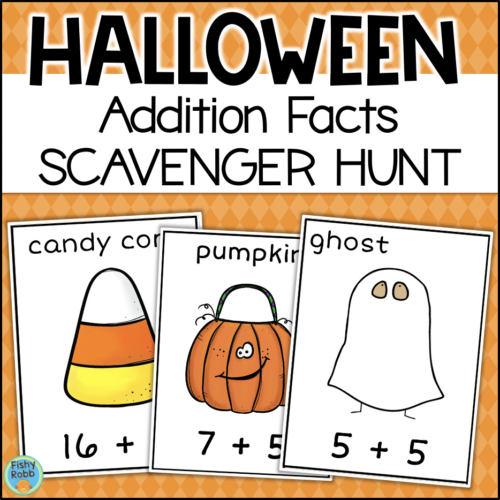\$2.00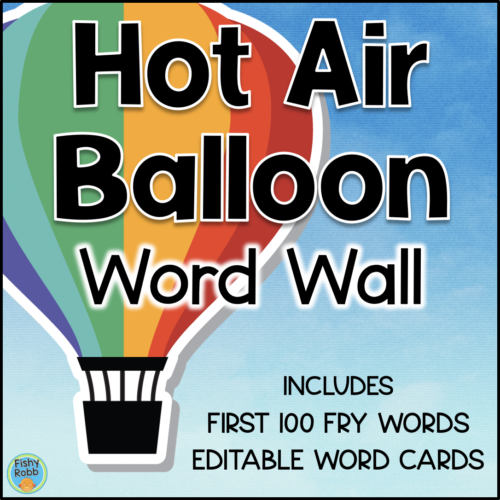\$3.00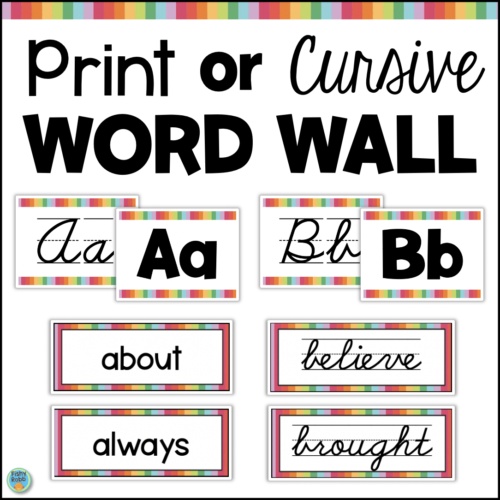\$3.50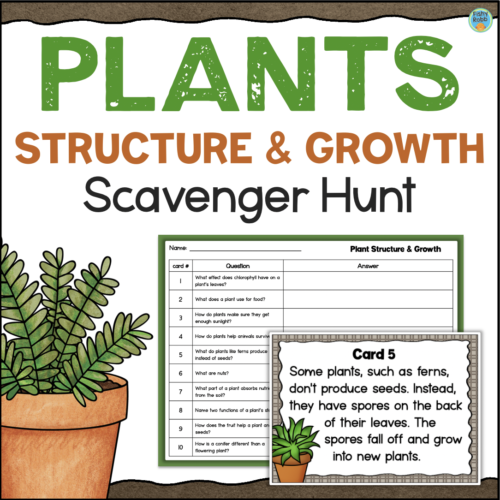\$3.00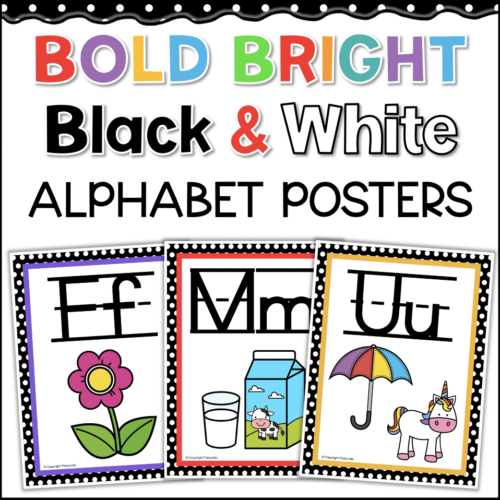\$3.00\$4.95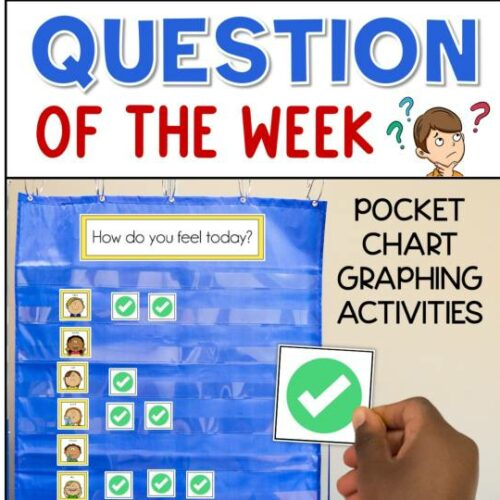\$4.75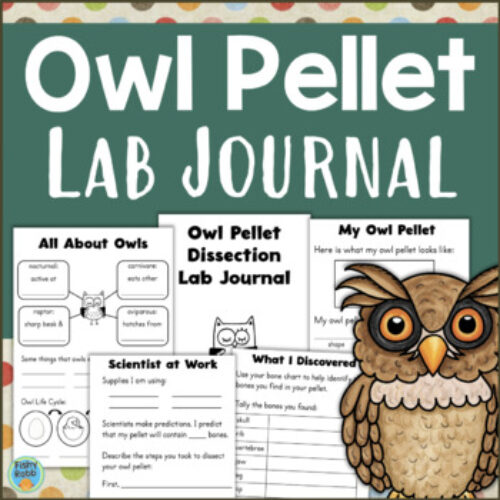\$2.25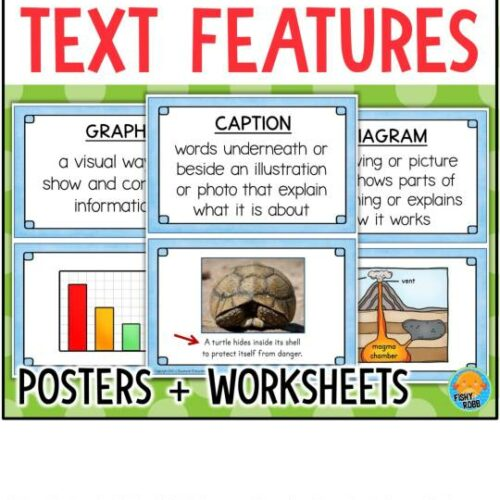\$4.25\$3.00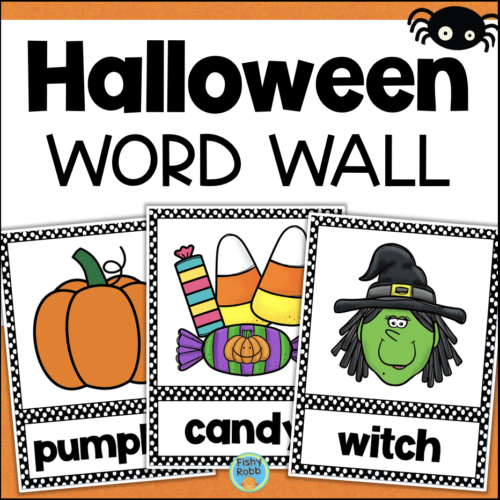\$2.50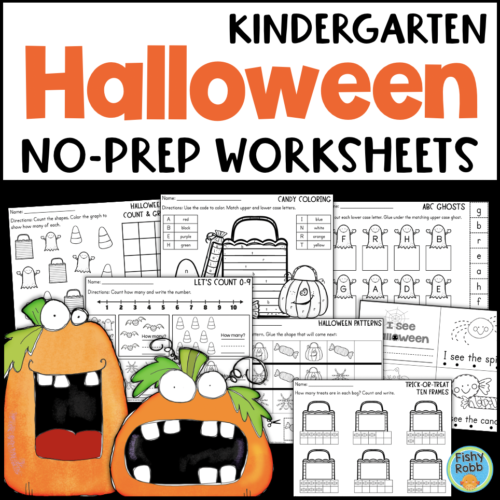\$3.00

Shop | Product

Janet Ecochardt
\$2.50